# Fraction Standards 4th-Grade: Sample Questions

4.NF.B.3A - 4.3E | Solve Addition with Equal Denominators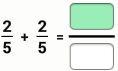Solve

Use your toolkit to model as needed

4.NF.B.3A - 4.3E | Model Addition with Equal Denominators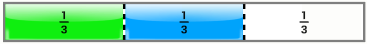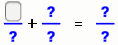Write an equation described by the model

4.NF.B.3A - 4.3E | Solve Subtraction with Equal Denominators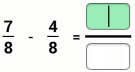Solve

Use your toolkit to model as needed

4.NF.B.3A - 4.3E | Model Subtraction with Equal Denominators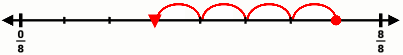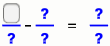Write an equation that matches the model.

## Benchmark & Decompose

4.NF.B.3X - 4.3F | Evaluate the reasonableness of sums and differences of fractions using benchmark fractions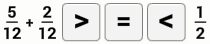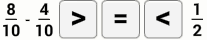Use your toolkit to model as needed

4.NF.B.3B - 4.3B | Decompose a fraction in more than one way into a sum of fractions

Decompose 5/8 into unit fractions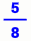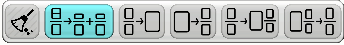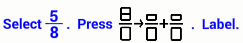What fraction of the race has she completed?

## Mixed Number Vocabulary & Conversion

4.NF.3C - 4.3E | Mixed Number Terminology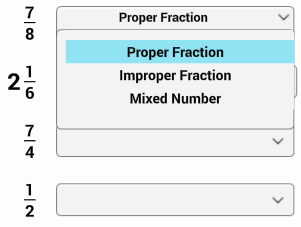Label each fraction type

4.NF.3D - 4.3E | Convert Improper Fraction to a Mixed Number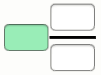Write 23/10 as a mixed fraction

4.NF.3D - 4.3E | Convert Improper Fraction to a Mixed Number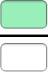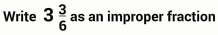4.NF.B.3D - 4.3E | Problem Solving with Fraction Addition

Jeni ate 6 tacos.

Kade ate 2 tacos and Kami ate 2 tacos.What fraction of ALL the tacos

Use your toolkit to model all the tacos.

4.NF.B.3D - 4.3E | Problem Solving with Fraction Subtraction

Felicity and Barry cleaned 10/12 of the windows.

Felicity cleaned 6 of the 12 windowsWhat fraction did barry clean?

Use your toolkit to model it.

4.NF.B.3X - 4.3F | Problem Solving with Benchmark Fractions

Peter ate half a pizza.

Wendy ate 4/12 of a pizza for breakfast

and 3/12 for lunch.

Peter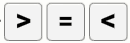Wendy

Who ate more pizza?

4.NF.B.3B - 4.3B | Problem Solving with Decomposing Fractions

Bonnie had a box of 12 chocolates. 11 are left.

Bonnie wants to give some to Clyde.Write two DIFFERENT ways Bonnie and Clyde

could split the fraction of remaining chocolates.

Model both in your toolkit as needed

4.NF.B.3C - 4.3E | Solve Addition with Mixed Fractions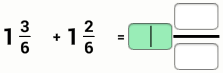Solve

Use your toolkit to model as needed

4.NF.B.3C - 4.3E | Solve Addition with Mixed Fractions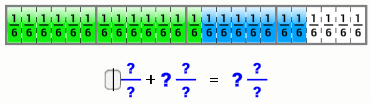Write an equation described by the model

4.NF.B.3C - 4.3E | Solve Subtraction with Mixed Fractions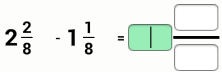Solve

Use your toolkit to model as needed

4.NF.B.3C - 4.3E | Model Subtraction with Mixed Fractions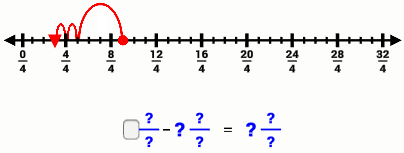Write an equation described by the model

## Mixed Addition & Subtraction—Problem Solving

4.NF.B.3D - 4.3E | Addition Problem Solving with Mixed Fractions

Mrs. Owen split 3 candy bars among 5 students.

Each student ate 1/2 of a candy bar.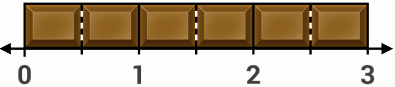What fraction of candy bar did the students eat total?

4.NF.B.3D - 4.3E | Addition Problem Solving with Mixed Fractions

Yasmine ate 3 1/4 hot dogs. Carlos ate 1 3/4.How many hot dogs did they eat combined?

4.NF.B.3D - 4.3E | Subtraction Problem Solving with Mixed Fractions

Rosie ran 2 3/12 miles and Eduardo ran 1 1/12How much farther did Rosie run than Eduardo?

## Compare Fractions with Different Denominators

4.NF.2 - 4.3D | Compare to Benchmark Fractions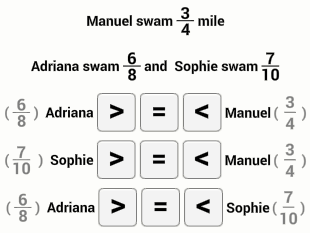4.NF.1 - 4.3D | Create Equivalent Fractions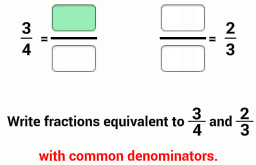4.NF.2 - 4.3D | Compare Different DenominatorsCompare

4.NF.2 - 4.3D | Story Problem: Compare Different Denominators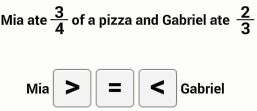Who ate more?

## Multiply Fractions

4.NF.4A - 5.3i | Multiply Unit FractionsSolve

4.NF.4B1 - 5.3i | Multiply Non-Unit Fractions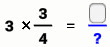Solve

4.NF.4B - 5.3i | Model Fraction Multiplication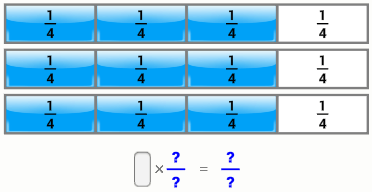Write an equation that represents the model

4.NF.4C - 5.3i | Story Problem Fraction Multiplication

Kim ate 3/4 cup of yogurt each day for 3 daysHow many cups of yogurt did Kim eat total?

Model in your toolkit as needed